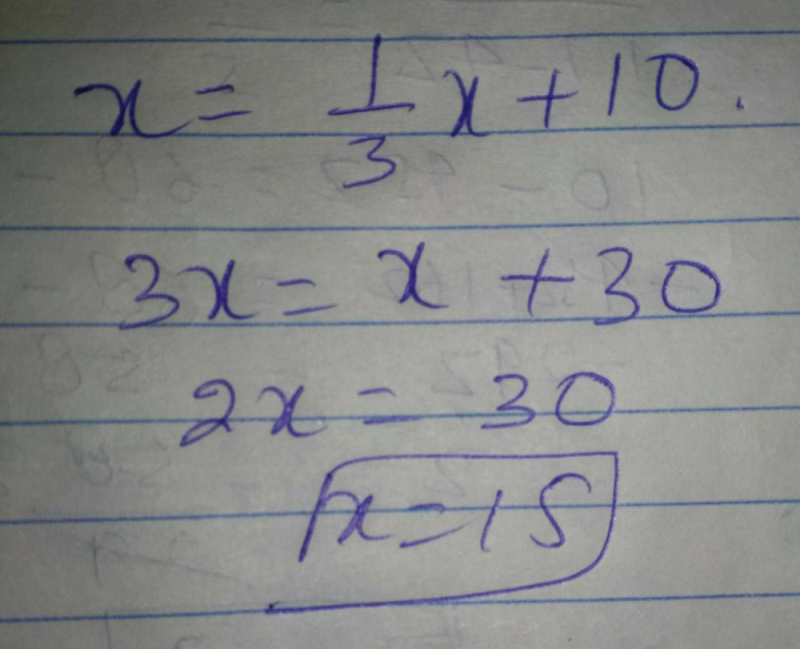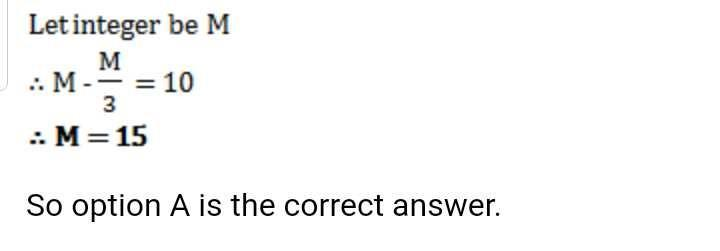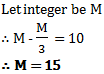Courses

# An integer is 10 more than its one-third part. The integer isa)15b)12c)22d)43Correct answer is option 'A'. Can you explain this answer? Related Test: Test: Integers - 1

## Class 7 Question

By Shraddha Satardekar · Sep 08, 2019 ·Class 7
15

Harshit Poddar answered Feb 28, 2020

15

Priya Verma answered May 07, 2020
because,
x = 1/3x + 10
3x = x + 30
3x - x = 30
2x = 30
Now, x= 30/2
=15

Payal Mittal answered Sep 08, 2019Kartik Kumar answered Apr 19, 2020
Potty

The answer is 15 option A

प्यारे लाल answered May 01, 2020

Pushpa Insan answered Apr 19, 2020

Ritika Nayak answered Sep 21, 2019
A

Syna Sharma answered Apr 03, 2020
1/3 of 15 is 5 so 5+10=15

Hasinee Reddy answered 4 weeks ago

Balram Kumar answered 3 weeks ago
Yes the right option is a

Mohanbabu Babu answered 4 days ago
Because,x=1/3×+10 3× + 30 3× - × 30 2×=30 Now, x=30/2=15

Yasmin Shaikh answered Apr 16, 2020
B 15

Aish Gzz answered 4 weeks ago

Singh Priyanka answered 6 days ago
Let the number be x
then 10+ x/3 = x
so 10 = x - x/3
thus 10 = 2x/3
so x = 10 ÷ 2/3
so x = 10 × 3/2
which means x = 15

Subhi Prajapati answered 3 weeks ago

Drama Queen answered Apr 08, 2020

Jawan Singh answered May 07, 2020
22

Jagjsonueet Singh answered Apr 26, 2020
15 is the anwser

Prakash Balannavar answered Apr 11, 2020
15

x=1/3 x +10
3x=x+30
2x=30
x=15

Swati Durve answered Apr 21, 2020
12

Hema Amrutha answered Aug 30, 2019

Sanjeev Jain answered Apr 01, 2020
Correct and is 15

Lalith Togalla answered Apr 13, 2020
The answer is 15 because a third of 15 is 5. and obviously 5 is 10 less than 15.

Anjum Akhtar answered 4 weeks ago

Nilakshi Chowdhury answered 4 weeks ago

Wahid Khan answered Nov 11, 2019Raju Kandharkar answered 2 weeks ago
(15.)

Akshita Suyal answered May 05, 2020

Mamta Jagota answered Apr 10, 2020
A)15

Mannat Mannat answered Apr 10, 2020
15
1/3 of 15 is 5
so 5+10= 15

Manoj Garg answered Apr 16, 2020

15 becauce it one-third part is 5 and 15 is 10 more than it

Kunti Singh Patel answered Apr 24, 2020
×=1_3×+10

3×=×+30

2×=30

×=15

Mahender Verma answered May 07, 2020
Check right I don't

Kinjal Saha answered Apr 13, 2020

Nandini Bhavar answered 3 weeks ago
Hi

Prakash Patange answered May 05, 2020
Ans 15

Urvi Patel answered Apr 29, 2020
15 .

Sahil Khatri answered 5 days ago
1/3 of 15 is 5 so 10+5=15

Rekha Prajapati answered 3 weeks ago
Ans is 15

Anand Raj answered Apr 17, 2020
B

Nandan Chowdbury answered 4 weeks ago
15.

Vijay Pandey answered Mar 23, 2020
V goog

Purvi Lohat answered Apr 26, 2020
Option - A 15

Saurav Rawat answered Aug 30, 2019So option A is the correct answer.

Rishabh Pal answered Apr 03, 2020
15

Vivek Shyamsukha answered Apr 01, 2020
Let the integer be x
x+1/3=10
2x/3=10
2x=10×3
2x=30
x=30/2
x=15

Ajeet Kumar answered Apr 25, 2020

Anavi Bhatt answered 2 weeks ago
Answer is 15 because one third of 15 is 5 and 5+10=15.

option b is incorrect as one third of 12 is 4 and 4+12is unequal to 12.

option c is incorrect as one third of 22 is 7.3 and 7.3+10 is unequal to 22.

option d is incorrect as one third of 43 is 14.3 and 14.3+10 is unequal to 43.

Virendra Verma answered 2 weeks ago
Answer is 15 Because One integer is 5 means 5+5+5=15

Mastermind Of All Subjec answered 3 weeks ago
Correct answer is 15 because the 1/3 of 15 is 5 then 5 is added to 10 and the answer is 15

Dhir Bhandari answered Apr 12, 2020
Let the integer be x

Now, x-x/3= 10
So, If you choose 15 as 'x' then,
15-5=10
Or if you take 12 as 'x' then,
12-4=8 not 10
If you take 22 as 'x' then,
22-7.3= 14.7 not 10
If you take 43 as 'x' then,
43-14.3=28.7 not 10

Priyanshi Dhote answered Apr 27, 2020
15 maybe

Dayaan Ali answered Apr 22, 2020
15 is correct ans

Sehrish Bilal answered May 04, 2020
Letno be x
it's 1/3=x/3
then x-x/3=10
(3x-x) ÷3=10
2x=30
x=30/2
=15

Neelu Gupta answered Sep 11, 2019

Mukesh Tiwari answered Apr 21, 2020
Sorry

Ayush Kumar answered Apr 19, 2020
15
let mean integer -y
yx1/3+10=y
then, 1y/3+10=y
now, 1y/3=y-10
now, y=3y-30
now, y-3y=-30
now,. -2y=-30
now,. +2y=+30
now, y=30/2 =>15

Yaahika Solanki answered May 07, 2020
Yes

Proy Karmakar answered 2 weeks ago
I am sure and the answer is 15

Aman Kumar answered Apr 16, 2020

Mandeep Kumar answered 4 weeks ago
Bbbb

Natarajan Raju answered May 04, 2020
The correct answer is a) 15

First of all 1/3 of 15 is 5 then so 5 + 10=15

Arvind Singh answered 2 weeks ago
Good

Raj Kumar Singh answered 2 weeks ago
A)15